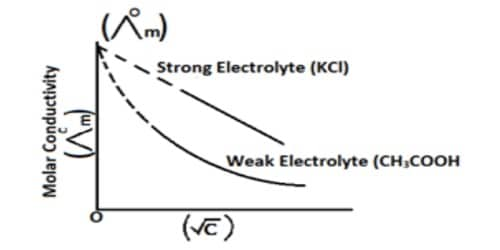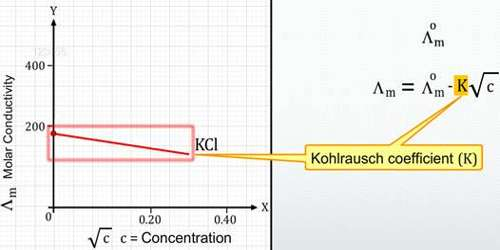# Debye-Huckel-Onsager Theory

Debye-Huckel-Onsager Theory

A theory providing quantitative results for the conductivity of ions in dilute solutions of strong electrolytes, which enables the Kohlrausch equation to be derived. Based on his analysis of the data available and his own experimental results the following empirical equation relating molar conductance (he was using equivalent conductance) with concentration was put forward by Kohlrausch-

Λ = Λ0 – A√C … … … (1)

where A is a constant. The constant A is characteristic of the electrolyte. The first satisfactory theoretical interpretation of the equation, however, was given in 1920 by Debye, Huckel, and Onsager, and the constant A in Kohlrausch’s equation became meaningful. This theory is providing quantitative results for the conductivity of ions in dilute solutions of strong electrolytes, which enables the Kohlrausch equation to be derived.

The Debye-Hückel-Onsager theory uses similar assumptions and approximations as the Debye-Hückel theory and is also limited to very dilute solutions (generally less than 10-3 M) for which there is good agreement between theory and experiment.Development of the study of crystals by X-rays and the electronic theory of valency led to the view that when a salt, strong acid a strong base is dissolved in water these are completely ionized. In the Debye-Huckel-Onsager theory, this is accepted as a fact and the increase of molar conductance with dilution is explained in terms of decreasing inter-ionic attraction at low concentration. A solution is defined as a homogeneous mixture of two or more components existing in a single phase. Chemists, therefore, have studied the processes involved in solution chemistry in order to further the understanding of the solution chemistry in nature.

Debye-Huckel-Onsager Theory is based on the following Assumptions;

• Electrolytes completely dissociate into ions in solution. The solute is completely dissociated; it is a strong electrolyte. Strong electrolytes are completely dissociated into ions.
• Due to Coulornbic forces between the charges of the ions, they do not behave like molecules in their transport and thermodynamic properties.
• Since the solution as a whole is neutral, the total number of positive charges must be equal to the total number of negative charges. Solutions of Electrolytes are very dilute, on the order of 0.01 M.
• It was assumed that the electric field causes the charge cloud to be distorted away from spherical symmetry.
• A given ion will have more ions of the opposite sign close to it than ions of the same sign. This cluster of ions is called the ionic atmosphere. Each ion is surrounded by ions of the opposite charge, on average.
• The solvent plays no role other than providing a medium of stable comparative permittivity (dielectric constant).
• Ions are spherical and are not polarized by the surrounding electric field. Solvation of ions is ignored except insofar as it determines the effective sizes of the ions.
• There is no electrostriction.